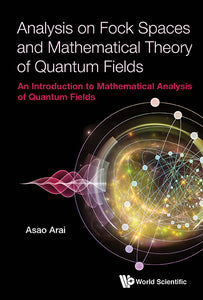# Analysis On Fock Spaces And Mathematical Theory Of Quantum Fields: An Introduction To Mathematical Analysis Of Quantum Fields

Asao Arai

Format: Print Book

ISBN: 9789813207110

• \$222.10
Unit price per
Tax included.

This book provides a comprehensive introduction to Fock space theory and its applications to mathematical quantum field theory. The first half of the book, Part I, is devoted to detailed descriptions of analysis on abstract Fock spaces (full Fock space, boson Fock space, fermion Fock space and boson-fermion Fock space). It includes the mathematics of second quantization, representation theory of canonical commutation relations and canonical anti-commutation relations, Bogoliubov transformations, infinite-dimensional Dirac operators and supersymmetric quantum field in an abstract form. The second half of the book, Part II, covers applications of the mathematical theories in Part I to quantum field theory. Four kinds of free quantum fields are constructed and detailed analyses are made. A simple interacting quantum field model, called the van Hove model, is fully analyzed in an abstract form. Moreover, a list of interacting quantum field models is presented and a short description to each model is given.To graduate students in mathematics or physics who are interested in the mathematical aspects of quantum field theory, this book is a good introductory text. It is also well suited for self-study and will provide readers a firm foundation of knowledge and mathematical techniques for reading more advanced books and current research articles in the field of mathematical analysis on quantum fields. Also, numerous problems are added to aid readers to develop a deeper understanding of the field.

Format: Hardcover
No of Pages: 892
Imprint: World Scientific
Publication date: 20171220